# Test: TCP/ UDP & Sockets

## 20 Questions MCQ Test GATE Computer Science Engineering(CSE) 2023 Mock Test Series | Test: TCP/ UDP & Sockets

Description
Attempt Test: TCP/ UDP & Sockets | 20 questions in 60 minutes | Mock test for Computer Science Engineering (CSE) preparation | Free important questions MCQ to study GATE Computer Science Engineering(CSE) 2023 Mock Test Series for Computer Science Engineering (CSE) Exam | Download free PDF with solutions
QUESTION: 1

### Host A is sending data to host B over a full duplex link. A and B are using the sliding window protocol for flow control. The send and receive window sizes are 5 packets each. Data packets (sent only from A to B) are all 1000 bytes long and the transmission time for such a packet is 50 μs. Acknowledgement packets (sent only from B to A) are very small and require negligible transmission time. The propagation delay over the link is 200 μs. What is the maximum achievable throughput in this communication?

Solution:

We need the maximum throughput, and for that we need to send as much data as possible to fully utilize the bandwidth.
so, maximum packets that can be sent = 1 + 2a = 9(after calculation) for 100% efficiency.
But we have a window size of 5 only, so we can send only 5 packets at max.
Efficiency = 5/9
Now, A/Q, Bandwidth of the channel (BW) = L/Tt = 1000 / (50*10^-6) = 20*10^6 bytes/sec.
So, max. throughput achievable = Efficiency * BW = 5/9 * 20*10^6 = 11.11 * 10^6 bytes/sec. (B)

QUESTION: 2

### In a sliding window ARQ scheme, the transmitter's window size is N and the receiver's window size is M. The minimumnumber of distinct sequence numbers required to ensure correct operation of the ARQ scheme is

Solution:

C) M+N
Because Ws+Wr ≤ Sequence numbers (as the maximum number of unacknowledged packets at sender will be Ws and at the receiver it will be Wr, similar to the sequence numbering in Selective Repeat)
where Ws is size of sender window and Wr is receiver window's size.

QUESTION: 3

### A 20 Kbps satellite link has a propagation delay of 400 ms. The transmitter employs the "go back n ARQ" scheme with n setto 10. Assuming that each frame is 100 bytes long, what is the maximum data rate possible?

Solution:

Transmission TIme = 100*8 bits/20 Kbps = 40 ms
Propagation Time = 400 ms
Efficiency = Window Size*Transmission Time/(Transmission Time + 2*Propagation Time) = 10*40/(40+2*400) = .476
Maximum Data Rate = .476*20 Kbps = 9.52 Kbps which is close to option B.

QUESTION: 4

Suppose that the maximum transmit window size for a TCP connection is 12000 bytes. Each packet consists of 2000 bytes.At some point of time, the connection is in slow-start phase with a current transmit window of 4000 bytes. Subsequently,the transmitter receives two acknowledgements. Assume that no packets are lost and there are no time-outs. What is themaximum possible value of the current transmit window?

Solution:

In slow-start phase, for each ACK, the sender increases the current transmit window by Maximum Segment Size (MSS). In
the question it is given a packet consists of 2000 bytes and that can be taken as MSS. So, after two ACKs, current transmit
window
= 4000 + 2000 + 2000
= 8000

QUESTION: 5

The maximum window size for data transmission using the selective reject protocol with frame sequence numbers is:

Solution:

ans b)
In selective reject protocol, the maximum window size must be half the sequence number space = 2n/2 = 2n-1.
For Go-back n, the maximum window size can be 2n-1

QUESTION: 6

Station A uses 32 byte packets to transmit messages to Station B using a sliding window protocol. The round trip delaybetween A and B is 80 milliseconds and the bottleneck bandwidth on the path between A and B is 128 kbps. What isthe optimal window size that A should use?

Solution:

Round Trip Time = 80ms
Frame size = 32
8 bits
Bandwidth = 128kbps
Transmission Time =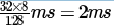Let
n be the window size.
Utilization =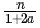where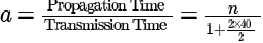For maximum utilization:
n = 41 which is close to option (B).

QUESTION: 7

Station A needs to send a message consisting of 9 packets to Station B using a sliding window (window size 3) and go-back near or control strategy. All packets are ready and immediately available for transmission. If every 5 th packet that A transmits gets lost (but no acks from B ever get lost), then what is the number of packets that A will transmit for sendingthe message to B?

Solution:

Since all packets are ready initially itself, we can assume a time out is detected after all possible packets are sent. So, the sending happens as shown in figure (I draw the figure assuming 10 packets. For 9 packets answer will be 16).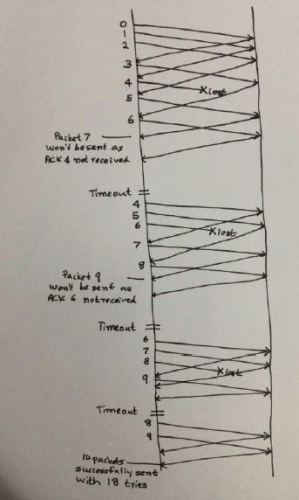QUESTION: 8

Suppose that it takes 1 unit of time to transmit a packet (of fixed size) on a communication link. The link layer uses a window flow control protocol with a window size of N packets. Each packet causes an ack or a nak to be generated by the receiver, and ack/nak transmission times are negligible. Further, the round trip time on the link is equal to N units. Consider time i > N. If only acks have been received till time i(no naks), then the goodput evaluated at the transmitter at time i (in packets per unit time) is

Solution:

In computer networks, goodput is the application level throughput, i.e. the number of useful information bits delivered by
the network to a certain destination per unit of time. (From wikipedia).
So, successful delivery of packet can be assured if ACK has been received for it.
So till time 'i' we would have transmitted 'i' packets but only (i-N) can be acknowledged as minimum time for a packet to
get Acknowledged is N (since RTT is N which is equal to the window size, there is no waiting time for the sender).
So successfully delivered packets = (i-N)
Time for transmission = i
Goodput = Successfully delivered data/ Time = (i-N)/i = 1- N/i
Therefore (A)

QUESTION: 9

The distance between two stations M and N is L kilometers. All frames are K bits long. The propagation delay per kilometer is t seconds. Let R bits/second be the channel capacity. Assuming that the processing delay is negligible, the minimum number of bits for the sequence number field in a frame for maximum utilization, when the sliding window protocol is used, is:

Solution:

for maximum utilization η = 1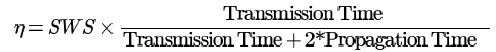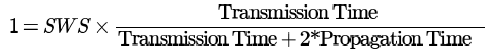so,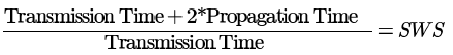gives the number of packets that are sent. It means that it is the Sender's Window Size.
But we also know that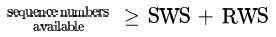so, to get the minimum number of bits needed to represent the sequence numbers we should consider what protocols are in use.
if GBN ARQ: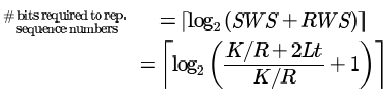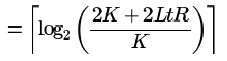if Selective Repeat ARQ :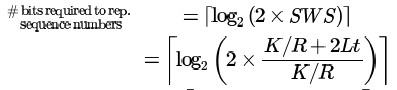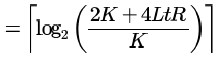QUESTION: 10

A 1 Mbps satellite link connects two ground stations. The altitude of the satellite is 36,504 km and speed of the signal is 3 ×108 m/s. What should be the packet size for a channel utilization of 25% for a satellite link using go-back-127 sliding window protocol? Assume that the acknowledgment packets are negligible in size and that there are no errors during communication.

Solution:

Distance from Station A to Satellite = 36504 x 103 m

Time to reach satellite =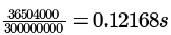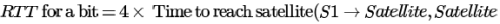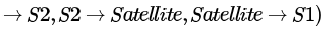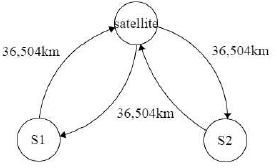Efficiency is the ratio of the amount of data sent to the maximum amount of data that could be sent. Let X be the packet size.
In Go-Back-N, within RTT we can sent n packets. So, useful data is nxX, where X is the packet size. Now, before we can sent another packet ACK must reach back. Time for this is transmission time for a packet (other packets are pipelined and we care only for first ACK), and RTT for a bit (propagation times for the packet + propagation time for ACK +
transmission time for ACK - neglected as per question)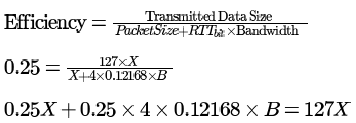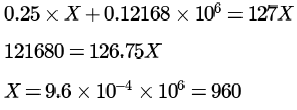Packet Size = 960 bits = 120 Bytes
so option (A)

QUESTION: 11

Frames of 1000 bits are sent over a 10bps duplex link between two hosts. The propagation time is 25ms. Frames are to be transmitted into this link to maximally pack them in transit (within the link).What is the minimum number of bits (I) that will be required to represent the sequence numbers distinctly? Assume that no time gap needs to be given between transmission of two frames.

Solution:

Bandwidth won't be halved in full duplex.
Propagation time is given as 25 ms.
Bandwidth = 106 bps.
So, to fully utilize the channel, we must send 106 bits into the channel in a second, which will be 1000 frames per second as each frame is 1000 bits. Now, since the propagation time is 25 ms, to fully pack the link we need to send at least 1000 * 25 * 10-3 = 25 frames. So, we need ⌈log2 25⌉ = 5 bits.

QUESTION: 12

The subnet mask for a particular network is 255.255.31.0. Which of the following pairs of IP addresses could belong to this network?

Solution:

A and C are not the answers as the second byte of IP differs and subnet mast has 255 for second byte.
Consider B, (& for bitwise AND)
10.35.28.2 & 255.255.31.0 = 10.35.28.0 (28 = 111002)
10.35.29.4 & 255.255.31.0 = 10.35.29.0 (29 = 111112)
So, we get different subnet numbers
Consider D.
128.8.129.43 & 255.255.31.0 = 128.8.1.0 (129 = 100000012)
128.8.161.55 & 255.255.31.0 = 128.8.1.0 (161 = 101000012)
The subnet number matches. So, D is the answer.

QUESTION: 13

The routing table of a router is shown below: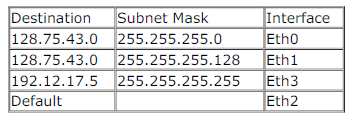On which interface will the router forward packets addressed to destinations 128.75.43.16 and 192.12.17.10 respectively?

Solution:

For 1st packet,
(128.75.43.16) && (255.255.255.0) = (128.75.43.0) since {16 && 0 = 0}, as well as
(128.75.43.16) && (255.255.255.128) = (128.75.43.0) since {16 && 128 = 0}.
Now, since both these subnet masks are producing the same Network ID, hence The one with greater number of ones will
be selected, and the packet will be forwarded there. Hence packet 1 will be forwarded to Eth1.
For 2nd packet,
(192.12.17.10) when anded with each of the subnet masks does not match with any of the network ID, since:
(192.12.17.10) && (255.255.255.0) = (192.12.17.0) {Does not match with any of the network addresses}
(192.12.17.10) && (255.255.255.128) = (192.12.17.0) {Does not match with any of the network addresses}
(192.12.17.10) && (255.255.255.255) = (192.12.17.10) {Does not match with any of the network addresses}
Hence, Default interface must be selected for packet 2, i.e Interface Eth2.

QUESTION: 14

A subnet has been assigned a subnet mask of 255.255.255.192. What is the maximum number of hosts that can belong tothis subnet?

Solution:

C is answer since you have 6 zeroes so u can make 64-2 hosts

QUESTION: 15

An organization has a class B network and wishes to form subnets for 64 departments. The subnet mask would be:

Solution:

to form subnet for 64 departments we need 6 continuous bit and the value of 11111100 = 252. organization has class B network so subnet mask would be 255.255.252.0

QUESTION: 16

Identify the correct sequence in which the following packets are transmitted on the network by a host when a browserrequests a webpage from a remote server, assuming that the host has just been restarted.

Solution:

Here
FIrst you send DNS request to get IP address. Then you establish connection with IP of google using TCP. Finally you start
talking in HTTP !

QUESTION: 17

Which one of the following statements is FALSE?

Solution:

Option B: "Sequence numbers allow receivers to discard duplicate packets and properly sequence reordered packets."
Option C: "When congestion is detected, the transmitter decreases the transmission rate by a multiplicative factor; for example, cut the congestion window in half after loss." (Additive Increase/multiplicative
decrease)
Option D: "Acknowledgments allow senders to determine when to retransmit lost packets."

QUESTION: 18

In TCP, a unique sequence number is assigned to each

Solution:

a) it should be byte

QUESTION: 19

For a host machine that uses the token bucket algorithm for congestion control, the token bucket has a capacity of 1 megabyte and the maximum output rate is 20 mega bytes per second. Tokens arrive at a rate to sustain output at a rate of 10mega bytes per second. The token bucket is currently full and the machine needs to send 12 mega bytes of data _____________ seconds.

Solution:

Initially token bucket is full.
Rate at which it is emptying is (20-10) MBps.
Time taken to empty token bucket of 1 MB is 1/10 i.e 0.1 sec.
Data send in this time is 0.1 * 20 = 2 MB (rate at which bucket is emptying is different from rate at which data is send) .
Data left to send is 12 - 2 = 10 MB .
Now bucket is empty and rate of token arriving is less than that of going out so effective data speed will be 10 MBps.
Time to send remaining 10 MB will be 1 sec. So total time is 1+0.1= 1.1sec

QUESTION: 20

A computer on a 10 Mbps network is regulated by a token bucket. The token bucket is filled at a rate of 2 Mbps. It is initially filled to capacity with 16 Megabits. What is the maximum duration for which the computer can transmit at the full 10 Mbps?

Solution:

New tokens are added at the rate of r bits/sec which is
2Mbps in the given question.
Capacity of the token bucket (b) = 16 Mbits
Maximum possible transmission rate (M) = 10Mbps
So the maximum burst time = b/(M-r) = 16/(10-2) = 2 seconds
here is the animation for token bucket hope this will help us to understand the concept.Use Code STAYHOME200 and get INR 200 additional OFF Use Coupon Code